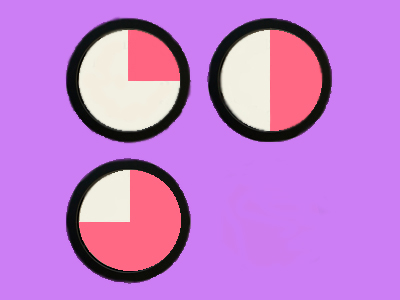14,  12,  34... what comes next in this sequence?

# Count up in Fractions

This Math quiz is called 'Count up in Fractions' and it has been written by teachers to help you if you are studying the subject at elementary school. Playing educational quizzes is an enjoyable way to learn if you are in the 3rd, 4th or 5th grade - aged 8 to 11.

It costs only \$12.50 per month to play this quiz and over 3,500 others that help you with your school work. You can subscribe on the page at Join Us

Being able to count up in fractions means understanding the value of a fraction and recognizing how many of each makes a whole. For example, when counting up in quarters, it will take four steps to reach one whole and 40 to reach 10. Children are expected to be able to count up to ten in fractions and this quiz will help them.

Try this quiz to see how well you do with counting up in fractions.

1.
What comes next: 24, 34, 1
44
114
2 14
1 34
Counting up in quarters means that after 1, it will be 1 + 14 more
2.
Which two numbers are next in this sequence?
214, 212, 234
3, 312
334, 4
244, 3
3, 314
Adding a quarter to 234 makes 3, and then adding another quarter makes 314
3.
Which two numbers come next in this sequence?
412, 5, 512, 6
522, 6
612, 7
6, 7
614, 7
The sequence is going up by 12 each time
4.
What comes next: 1, 112, 2, 212
2
312
3
222
Adding another half to 212 results in 3
5.
What is wrong with this sequence:
314, 324, 312, 334
334 is too large for the sequence
314 is in the wrong place
324 is the same as 312
There aren't enough numbers in the sequence
324has exactly the same value as 312 so shouldn't be there!
6.
What are the next three numbers in this sequence?
7, 714, 712
734, 744, 754
734, 8, 814
7, 812, 9
744, 8, 9
The sequence is going up by a quarter each time. 712 is the same as 724
7.
Which three numbers come next?
312, 334, 4
4, 5, 6
434, 5, 534
424, 434, 4 44
414, 412, 434
The numbers are one quarter more each time
8.
What is happening in this sequence:
214, 224, 234, 3
The numbers increase by 24 each time
The numbers increase by 12 each time
The numbers increase by 13 each time
The numbers increase by 14 each time
Each number is 14 more than the one before it
9.
Which two numbers are missing:
512, ___, 612, ___
6 and 7
5 and 6
7 and 8
6 and 9
The sequence is going up in halves
10.
Which two numbers are missing:
3, ___, 312, 334, 4, ___
324 and 412
314 and 4
414 and 514
314 and 414
Each number is one quarter more than the one before it
Author:  Angela Smith